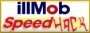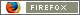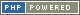### Bypass A Masterlock Combination

Have you ever had a Master Lock but forgot the combination? We all know the soda can method where youcreate a ‘shim’ in order to bypass the locking mechanism. Here is an easy tutorial on how to find a lost code. Caution: The newer Master Locks may or may not work. It is much more difficult to distinguish the difference between the different sticky numbers in the newer models.

1. Find the last number of the combination by finding the “sticky numbers”

1. Pull out the top loop of your Master Lock while turning the dial

2. There are about 12 spots where the Master Lock locks into place, these are the sticky numbers

3. There are 7 “sticky numbers” that lock in-between two number dashes, ignore these

4. There are 5 “sticky numbers” that lock in-between the half-way points between two numbers, record these numbers

1. One of these numbers’ last digit is different from the other four, keep this number, it is the last number in the combination

NOTE: The 5 sticky numbers might be difficult to figure out, make sure the correct sticky numbers follow these directions.

1. Find the first number in the combination by using “modulus 4”

1. Figure out the highest possible multiple of 4 with out exceeding the last number in the combination

2. Find the positive difference between the two numbers, this is the new number “x”

3. Add “x” to 4 to come up with the new number “y”, record “y”

4. Record “x”

5. Add 4 to “y” as many times as possible without exceeding 39, record these numbers

6. You should have recorded 10 numbers

7. These are the possible choices for the 1st number in the combination

1. Find the second number in the combination by following the applicable directions below:

1. If “x” is 0 than turn it into 2, this is the new “x”, proceed to direction #4

2. If “x” is 1 then turn it into 3, this is the new “x”, proceed to direction #4

3. If “x” is 2 then turn it into 0, this is the new “x”, proceed to direction #4

4. If “x” is 3 then turn it into 1, this is the new “x”, proceed to direction #4

1. Find the second number in the combination by using modulus 4

1. Add “x” to 4 to come up with the new number “y”, record “y”

2. Record “x”

3. Add 4 to “y” as many times as possible without exceeding 39, record these numbers

4. You should have recorded 10 numbers

5. These are the possible choices for the 2nd number in the combination

1. There are now 100 possible combinations out of the theoretical 64,000 combinations, write out the possibilities and try them out on the lock.

1. Relax and good luck

2. MAKE SURE YOU WRITE DOWN THE COMBINATIONS THAT YOU HAVE ALREADY TRIED SO YOU DON’T FORGET THE CORRECT COMBINATION WHEN YOU FIND IT!!!

Another math related method on discerning the combination can be found here

#### 2 Responses to ' Bypass A Masterlock Combination '

1. pablo said,

on February 28th, 2010 at 4:12 pm

so what is modulus 4? had it going until I reached that part

2. jdollar said,

on May 8th, 2010 at 6:48 am

If you can find both the first number and the last number in the 3-number combination you only have to try ten combinations.

4urnfo.com/lockpicking/find-the-first-number.htm

•